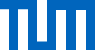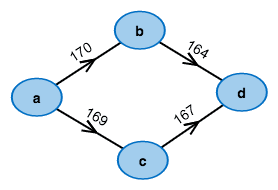What's the cheapest way from left to right?

# An informed search for the shortest path

You want to know, how to get from Munich to Frankfurt as fast as possible? Dijkstra's Algorithm can help you! But if you already know that Munich lies shouth of Frankfurt, this algorithm may be unnecessarily slow. Dijkstra's algorithm expands its search uniformly into all directions. It is, however, much faster to simply ignore most cities north of Frankfurt.

The A* algorithm was designed for these kinds of problems. It is similar to Dijkstra's algorithm, but its approach is much more goal-oriented. For the target node, Munich, it first computes an estimate of the shortest distance. Based on this estimate, it will achieve a fast computation

The A* algorithm can also compute the shortest distances between one city and all other cities. And the edges can describe costs, distances, or some other measure that is helpful for the user. It requires, however, that all edge costs (which is how we call the edge labels) must not be negative.

## Legend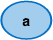NodeEdge with weight 50

## Which graph do you want to execute the algorithm on?

### Modify it to your desire:

• To create a node, make a double-click in the drawing area.
• To create an edge, first click on the output node and then click on the destination node.
• The edge weight is changed with a double-click on the edge.
• Right-clicking deletes edges and nodes.

A occured when reading from file: the contents:

## Legend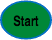Starting node from where the shortest path to the target is computed.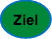Target node, towards whom the shortest path is computed.Node that has already been processed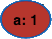Node being processed.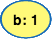Node in the priority queue.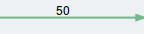"Predecessor edge" that is used by the shortest path to the node.

## Algorithm status

BEGIN

d(v) ← 0, parent(v) ← v

FOR i = 2,..,n DO

d(v[i]) ← ∞, parent(v[i]) ← NULL

h(v[i]) ← √(Σ(v[i].coord-ziel.coord)2)

queue.insert(v,0)

WHILE queue ≠ ∅ DO

u = queue.extractMin()

IF u == ziel DO RETURN "Path found!"

FOR ALL (u,w) ∈ E DO

dist ← d(u) + l(u,w)

IF w ∈ queue AND d(w) > dist DO

d(w) = dist, f = dist + h(u)

parent(w) = u

ELSE IF parent(w) == NULL THEN

d(w) = dist, f = dist + h(u)

parent(w) = u, queue.insert(w,f)

RETURN "Target unreachable"

END

### Priority Queue:

Your browser does not support HTML5 Canvas. Please use a modern browser, i.e.Mozilla Firefox

## Task is terminated if the tab is changed.

You can open another browser window for reading the description in parallel.

## LegendStarting node from where the shortest path to the target is computed.Target node, towards whom the shortest path is computed.Node that has already been processedNode being processed.Node in the priority queue."Predecessor edge" that is used by the shortest path to the node.

## Exercise: How does Dijkstra's algorithm work on a grid?

BEGIN

d(v) ← 0

FOR i = 2,..,n DO

d(v[i]) ← ∞, parent(v[i]) ← NULL

WHILE queue ≠ ∅ DO

u = queue.extractMin()

FOR ALL (u,w) ∈ E DO

dist ← d(u) + l(u,w)

IF w ∈ queue AND d(w) > dist DO

d(w) = dist, parent(w) = (u,w)

ELSE IF parent(w) == NULL THEN

d(w) = dist, parent(w) = (u,w)

queue.insert(w,dist)

END

## Task is terminated if the tab is changed.

You can open another browser window for reading the description in parallel.

## LegendStarting node from where the shortest path to the target is computed.Target node, towards whom the shortest path is computed.Node that has already been processedNode being processed.Node in the priority queue."Predecessor edge" that is used by the shortest path to the node.

## Exercise: How does the A* algorithm work on a grid?

BEGIN

d(v) ← 0, parent(v) ← v

FOR i = 2,..,n DO

d(v[i]) ← ∞, parent(v[i]) ← NULL

h(v[i]) ← √(Σ(v[i].coord-ziel.coord)2)

WHILE queue ≠ ∅ DO

u = queue.extractMin()

IF u == ziel DO RETURN "Path found"

FOR ALL (u,w) ∈ E DO

dist ← d(u) + l(u,w)

IF w ∈ queue AND d(w) > dist DO

d(w) = dist, f = dist + h(u)

parent(w) = u

ELSE IF parent(w) == NULL THEN

d(w) = dist, f = dist + h(u)

parent(w) = u, queue.insert(w,f)

RETURN "Target not reachable"

END

## Task is terminated if the tab is changed.

You can open another browser window for reading the description in parallel.